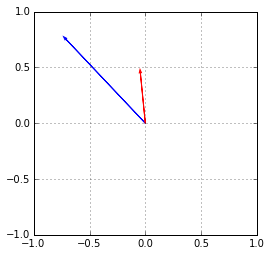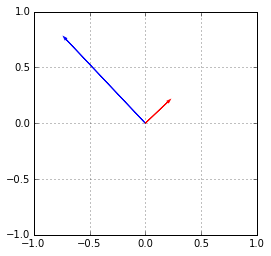# Orthogonalizing vectors

In :
import numpy as np
import matplotlib.pyplot as pt

In :
np.random.seed(13)
x = np.random.randn(2)
y = np.random.randn(2)

In :
pt.arrow(0, 0, x, x, color="blue")
pt.arrow(0, 0, y, y, color="red")
pt.xlim([-1, 1])
pt.ylim([-1, 1])
pt.gca().set_aspect("equal")
pt.grid()Are those orgonal? How would we find out?

In :
x.dot(y)

Out:
0.37226452785072001


Now use the formula to make ynew which is orthogonal to x:

In :
ynew = y - y.dot(x)/x.dot(x)*x

In :
pt.arrow(0, 0, x, x, color="blue")
pt.arrow(0, 0, ynew, ynew, color="red")
pt.xlim([-1, 1])
pt.ylim([-1, 1])
pt.gca().set_aspect("equal")
pt.grid()In []: